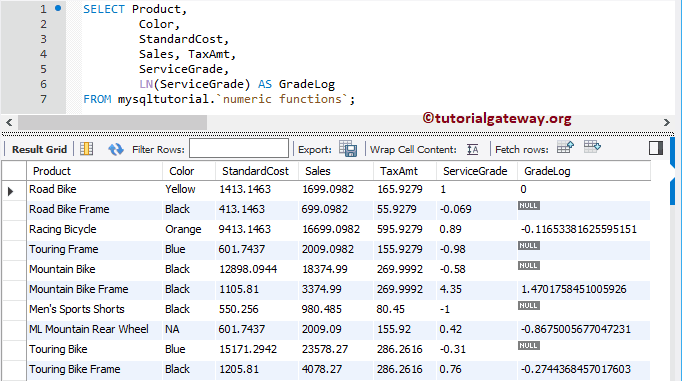# MySQL LN Function

How to use LN function find logarithmic values in MySQL using Command Prompt and Workbench with an example?. The LN is one of the Mathematical Functions, which is useful to return the natural logarithmic value of a given number with base E. The basic syntax of this is as shown below:

```SELECT LN (x)
FROM Source```

If x is less than or equal to 0.0E0, then the method returns the NULL value as output.

## MySQL LN Function Example 1

The LN function returns the natural logarithmic value of any numeric, and the following query shows multiple ways to use this.

```SELECT LN(2);

SELECT LN(0);

SELECT LN(-5);

SELECT LN(10);```

The MySQL LN function also allows you to find the logarithmic value of column data. To demonstrate this Mathematical method, we are going to use the below shown data.

In this MySQL example, we are going to find the logarithmic values for all the records present in the ServiceGrade column. And you can see the result.

```SELECT Product,
Color,
StandardCost,
Sales, TaxAmt,